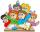# Clubhouse

There were only chairs and table in the clubhouse. Each chair had four legs, and the table was triple. Scouts came to the clubhouse. Everyone sat on their chair, two chairs were left unoccupied, and the number of legs in the room was 101. How many chairs were in the clubhouse?

Result

n =  17

#### Solution:

101 = 3+2*4 + (4+2)*(n-2)

6n = 102

n = 17

Calculated by our simple equation calculator.

Leave us a comment of example and its solution (i.e. if it is still somewhat unclear...):

Showing 0 comments:Be the first to comment!#### To solve this example are needed these knowledge from mathematics:

Do you have a linear equation or system of equations and looking for its solution? Or do you have quadratic equation?

## Next similar examples:

1. CagesHonza had three cages (black, silver, gold) and three animals (guinea pig, rat and puppy). There was one animal in each cage. The golden cage stood to the left of the black cage. The silver cage stood on the right of the guinea pig cage. The rat was in the
2. Write decimalsWrite in the decimal system the short and advanced form of these numbers: a) four thousand seventy-nine b) five hundred and one thousand six hundred and ten c) nine million twenty-six
3. FactoryIn the factory workers work in three shifts. In the first inning operates half of all employees in the second inning and a third in the third inning 200 employees. How many employees work at the factory?
4. Equation 29Solve next equation: 2 ( 2x + 3 ) = 8 ( 1 - x) -5 ( x -2 )
5. Last pageTwo consecutive sheets dropped out of the book. The sum of the numbers on the sides of the dropped sheets is 154. What is the number of the last page of the dropped sheets?
6. ClassroomIn a class are 32 pupils. Boys are 8 less than girls. How many boys and girls are in the classroom?
7. Seven timesWhich number seven times is just as higher as 27, how much is smaller than 29?
8. Unknown number 11That number increased by three equals three times itself?
9. Simple equationSolve the following simple equation: 2. (4x + 3) = 2-5. (1-x)
10. EquationSolve the equation: 1/2-2/8 = 1/10; Write the result as a decimal number.
11. Forest nurseryIn the forest nursery after winter, they found that 1/10 stems died out of them. For them, they land 193 new spruces. How many spruces are in the forest nursery?
12. Equation?
13. Find xSolve: if 2(x-1)=14, then x= (solve an equation with one unknown)
14. Roman numeralsWrite numbers written in Roman numerals as decimal.
15. If-then equationIf 5x - 17 = -x + 7, then x =
16. Unknown numberIdentify unknown number which 1/5 is 40 greater than one tenth of that number.
17. Addition of Roman numbersAdded together and write as decimal number: LXVII + MLXIV